# Visualising solid shapes Important Questions

In this page we have Visualising solid shapes Important Questions for Class 7 Maths Chapter 15 . Hope you like them and do not forget to like , social share and comment at the end of the page.
Question 1.
Fill in the blanks:-
i. Plane figure is also called_________________
ii. A solid shape that has only a curved surface is called a ________________
iii. A _______________ is a solid who bases are identical polygon faces and other faces are rectangles.
iv. A pyramid is a solid whose base is a flat __________________ figure and whose side faces are triangles having a common vertex outside the surface of the ______________
v. A triangular pyramid in which all faces are equal is called ______________________
vi. A    ________________ of a solid figure demonstrates how it is constructed by showing its sides, faces and vertices.
vii. To make a solid from a sheet of flat paper, we need to construct a ______________
viii. A line where two faces of a solid meet is called its ___________
ix. The term isometric refers to _________________________________
x. The below figure is the net of _____________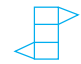xi. A triangular prism has __________ faces, __________ edges and __________ vertices.
xii.  A triangular pyramid has __________ faces, __________ edges and __________vertices.
Question 2.
What is Leonhard Euler’s rule?
Question 3.
Label the net.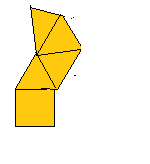Question 4.
Draw the net of a cube. Put the numbers 1 to 6 on the faces so that the numbers on opposite faces add to 7.
Question 5.
Name the different plane shape needed to draw the net of
a. a cube
b. a triangular prism
c. a triangular pyramid
d. a cylinder
Question 6.
The cross-section of diagonal cut of a cube creates which shape?
Question 7.
What cross-section would you get when you give a
a) Vertical cut
b) Horizontal cut to the following objects
ii. a dice
iiii. An unsharpened pencil
vi. A triangular prism

Question 8
Which of these two figure can be the net for tetrahedron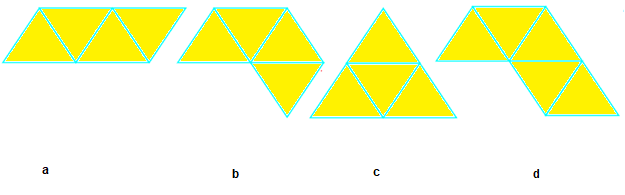Question 9
True and false
a. A pyramid has only one vertex
b. If the base of a pyramid is a square, it is called a square pyramid.
c. The solid shapes are of two-dimensional.
d. A net is a skeleton-outline of a solid that can be folded to make it.
e. All the faces, except the base of a square pyramid are triangular.

Question 10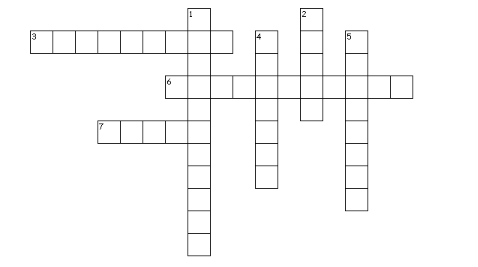Across
3. A sketch whre the measurements kept proportional
6. A pyramid with triangle base
7. the line segments of the skeleton of solid shape
Down
1. solid shapes are three-______
2. flat surfaces of solid shapes
4. it is the useful way to see how three-dimensional objects can be viewed in two dimensions
5. The corners of a solid shape

1.
i. 2d figure
ii. sphere
iii. Prsim
iv. rectangular, base
v, regular tetrahedron
vi. net
vii. net
viii. edge
ix. proportion to lenght /equal measure
x. trianglar prism
xi. 5,9,6
xii 4,6,4
2. $F + V = E + 2$
Where F,V,E denote number of faces, number of vertices and number of edges respectively of such solids. This is known as ‘EULER’s FORMULA
3. Square pyramid
4.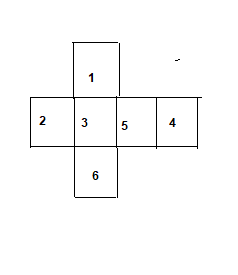5.
a. 6 squares
b. 2 triangle and 3 rectangle
c. 4 triangle
d. 2 circle and 1 rectangle
6. square
7.
Vertical , Horizontal
a. circle , circle
b. square, square
c. rectangle, circle
d. triangle, rectangle
8. a, c
9.
a. false
b. true
c. false
d. true
e. true
10.
i. dimensional
ii. faces
iii.isometric
v. vertices
vi. tetrahedron
vii. edges

• Worksheets